# ROM Conventional and Shared Bit Lines Structures

Make it to the Right and Larger Audience

Blog

# ROM Conventional and Shared Bit Lines Structures

Conventional structure of Read Only Memory (ROM) is one NMOS transistor per bit. In below diagram, WL is word line, BL is bit line, and Do is rom data out. To access the first word, WL0=1 so the corresponding NMOS transistors are closed (shorted). WL1=0 and other WLn=0 so the corresponding NMOS transistors are all open. If the transistor is connected to the vertical bit line (BL), BL is low since the pre-charged BL is discharged through the transistor. If not connected, BL stays high since no discharge path. Connected or not determines ROM content. The vertical Bit Line (BL) is also rom data out bit.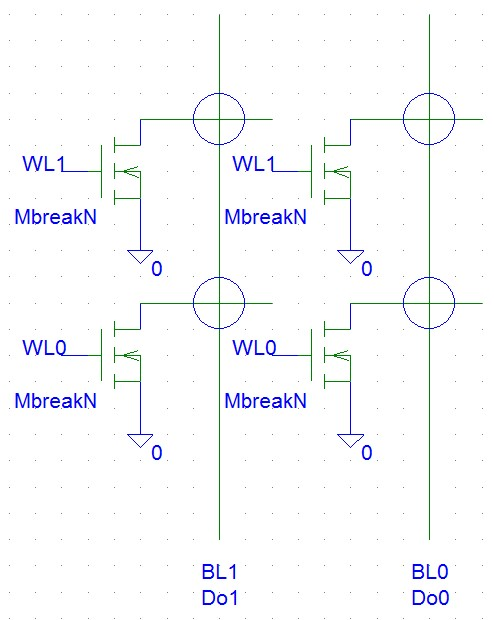Here is another illustration.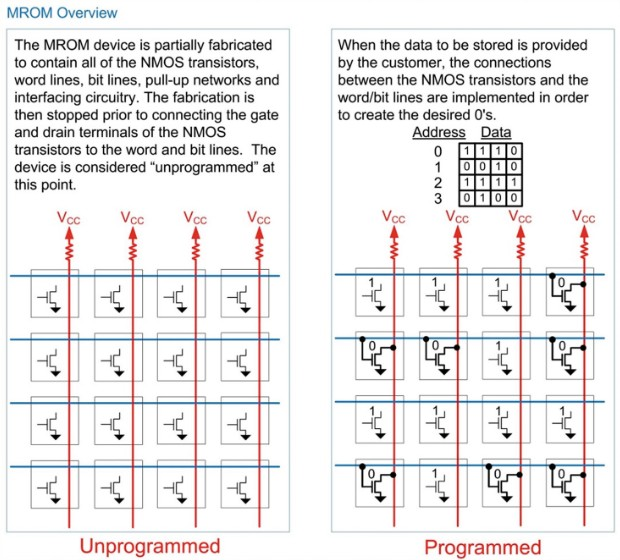Above conventional ROM is one transistor per bit. Is there a way to reduce number of transistors? Indeed it is possible. Below is the shared bit line structure for high density ROM.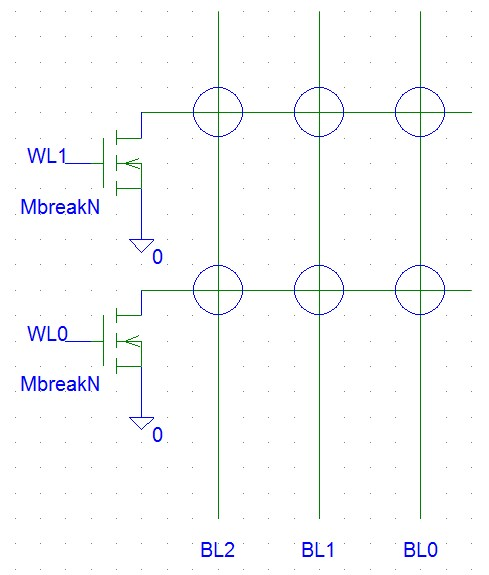As in conventional structure, when the first word is accessed, WL0=1 and other WLs are 0. We have 3 bit lines connected to one NMOS transistor. But these 3 bit lines represent ONLY two bits. Below is the bit mapping. Note only 4 combinations of BL[2:0] are used. For each combination, at most there is one 0 in BL[2:0].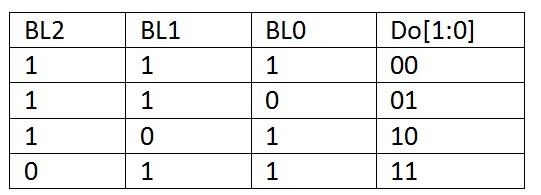The question is why other combinations of BL[2:0] are not used? Let’s say BL[2:0]=001 for word 1. It makes BL2 and BL1 shorted together and will affect other words. So in this shared bit line structure, each bit line can have at most one 0.

We can use 7 bit lines for three bits of data. Again, each bit line has at most one 0.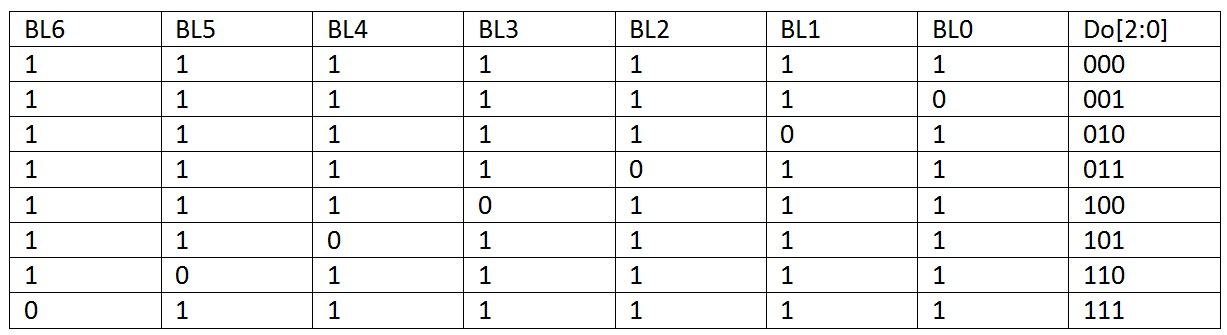Bit line uses metal layer connection so although transistor is saved metal layer usage increases.Author brief is empty
Groups:

Tags: